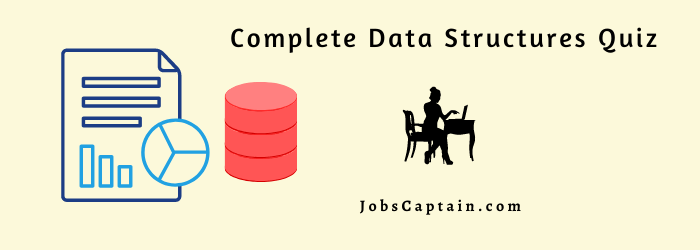Here you can find the latest Data Structures MCQ Questions with Answers. All quizzes on Computer Data Structures have answers available with pdf.

These are the selective and important questions of Data Structures.

Play MCQ Quiz

Data Structures Quiz – 1

Data Structures Quiz – 2

Advance Data Structure Quiz – 3

Advance Data Structure Quiz – 4

## Data Structures MCQ Questions and Answers – 1

Question 1: A _____________ refers to a single unit of values.

(A) data value

(B) attribute value

(C) data item

(D) elementary

(C) data item

Question 2: Data items that are divided into sub-items are called ___________.

(A) single items

(B) group items

(C) elementary items

(D) entity items

(B) group items

Question 3: ____________ of these best describes an array.

(A) A data structure that shows a hierarchical behavior

(B) Container of objects of similar types

(C) Container of objects of mixed types

(D) All of the above

(B) Container of objects of similar types

Question 4: In _____________ all the records contain the same data items with the same amount of space.

(A) variable-length records

(B) fixed-length records

(C) subscripted variable

(D) superscripted variable

(B) fixed-length records

Question 5: The logical or mathematical model of a particular organization of data is called a __________ ?

(A) data structure

(B) algorithms

(C) structure

(D) logic structure

(A) data structure

Question 6: Arrays are the best data structures for?

(A) relatively permanent collections of data

(B) the size of the structure and the data in the structure are constantly changing

(C) both of the above situation

(D) None of the above

(A) relatively permanent collections of data

Question 7: How do the nested calls of the function get managed?

(A) Through Queues

(B) Through Stacks

(C) Through Trees

(D) Through Graphs

(B) Through Stacks

Question 8: What is combining the records in two different sorted files into a single sorted file?

(A) Sorting

(B) Searching

(C) Listing

(D) Merging

(D) Merging

Question 9: In linear search algorithm the Worst case occurs when ____________.

(A) The item is somewhere in the middle of the array.

(B) The item is not in the array at all.

(C) The item is the last element in the array.

(D) The item is the last element in the array or is not there at all.

(D) The item is the last element in the array or is not there at all.

Question 10: The complexity of Binary search algorithm is ____________.

(A) O(n)

(B) O(log n )

(C) O(n2)

(D) O(n log n)

(B) O(log n )

Question 11: The complexity of Bubble sort algorithm is ____________.

(A) O(n)

(B) O(log n)

(C) O(n2)

(D) O(n log n)

(C) O(n2)

Question 12: In order traversal of binary search tree will produce _______________.

(A) unsorted list

(B) sorted list

(C) reverse of input

(D) none of these

(B) sorted list

Question 13: Sub algorithms fall into two basic categories: function sub algorithms and ____________ sub algorithms.

(A) procedure

(B) argument

(C) processor

(D) methods

(A) procedure

Question 14: Two main measures for the efficiency of an algorithm are ____________.

(A) Processor and memory

(B) Complexity and capacity

(C) Time and space

(D) Data and space

(C) Time and space

Question 15: New data are to be inserted into a data structure, but there is no available space; this situation is usually called ____________.

(A) Underflow

(B) Overflow

(C) Houseful

(D) Saturated

(B) Overflow

Question 16: Which of the following data structure is linear data structure?

(A) Tree

(B) Graph

(C) Array

(C) Array

Question 17: Which of the following is an example of a dynamic programming approach?

(A) Fibonacci Series

(B) Tower of Hanoi

(C) Dijkstra Shortest Path

(D) All of the above

(D) All of the above

Question 18: The memory address of the first element of an array is called _________.

Question 19: Which data structure allows deleting data elements from the front and inserting them at the rear?

(A) Stacks

(B) Queues

(C) Dequeues

(D) Binary search tree

(B) Queues

Question 20: Binary search algorithm cannot be applied to ________ concept.

(B) sorted binary trees

(C) sorted linear array

(D) pointer array

Question 21: Graph traversal is different from a tree traversal, because __________ .

(A) trees are not connected

(B) graphs may have loops

(C) trees have root

(D) None is true as tree is a subset of graph

Question 22: Linked lists are suitable for which of the following problems?

(A) Insertion sort

(B) Binary search

(D) dequeue

(B) Binary search

Question 23: Identify the data structure which allows deletions at both ends of the list but insertion at only one end ___________.

(A) Input-restricted dequeue

(B) Output-restricted dequeue

(C) Priority queues

(D) Data structure

(A) Input-restricted dequeue

Question 24: Which of the following data structure is non-linear type?

(A) Strings

(B) Lists

(C) Stacks

(D) Hierarchical

(D) Hierarchical

Question 25: To represent hierarchical relationship between elements, which data structure is suitable?

(A) Dequeue

(B) Priority

(C) Tree

(D) Binary tree

(C) Tree

Question 26: When does the ArrayIndexOutOfBoundsException occur?

(A) Compile-time

(B) Run-time

(C) Not an error

(D) None of the above

(B) Run-time

Question 27: When converting the binary tree into an extended binary tree, all the original nodes in binary tree are ___________.

(A) internal nodes on extended tree

(B) external nodes on extended tree

(C) vanished on extended tree

(D) post order traversal

(A) internal nodes on extended tree

Question 28: Which of the following conditions checks available free space in avail list?

(A) Avail=Top

(B) Null=Avail

(C) Avail=Null

(D) Avail=Max stack

(C) Avail=Null

Question 29: Which of the following sorting algorithm is of divide-and-conquer type?

(A) Bubble sort

(B) Insertion sort

(C) Quick sort

(D) Algorithm

(C) Quick sort

Question 30: STACK is also called as ______________.

(A) FIFO

(B) LIFO

(C) FOLI

(D) FOFI